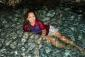# Differential Equationfind a general solution

dx/dy =[ -2x (csc 2y – 2x3 cos 2y)] / sec 2y

• Mathematics inside the configured delimiters is rendered by MathJax. The default math delimiters are $$...$$ and $...$ for displayed mathematics, and $...$ and $...$ for in-line mathematics.